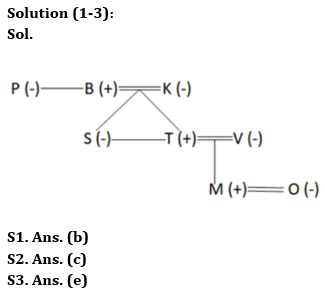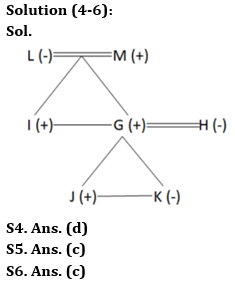Latest Banking jobs   »     »

# Reasoning Quiz For Bank of Baroda SO 2023 -03rd June

Directions (1-3): Study the information carefully and answer the questions accordingly.
There are eight members in a family with three married couples. P is the aunt of T who is the son of K. O is the daughter-in-law of V. B is the father of T and S who is unmarried. S is the sister-in-law of V. M is the grandson of K who does not have a sibling. P has no sisters.

Q1. Who is the husband of O?
(a) K
(b) M
(c) T
(d) B
(e) None of these

Q2. How M is related to B?
(a) Uncle
(b) Son
(c) Grandson
(d) Nephew
(e) None of these

Q3. Four from the following five are similar in a certain way and thus form a group. Find out the one who does not belong to that group?
(a) P
(b) K
(c) S
(d) O
(e) T

Directions (4-6): Study the information carefully and answer the questions accordingly.
There are seven members in a family. H is the daughter-in-law of L who is the grandmother of K’s brother. M is the father of two children in which one of them is married. G and H are married to each other. K is the sister of J. J is the only son of G whose brother is I.

Q4. How M is related to G?
(a) Brother
(b) Son
(c) Grandfather
(d) Father
(e) None of these

Q5. Who is the father of K?
(a) L
(b) M
(c) G
(d) I
(e) None of these

Q6. How many female members in the family?
(a) One
(b) Two
(c) Three
(d) None
(e) More than three

Directions (7-10): In the following questions assuming the given statement to be true, find which of the conclusion(s) among given conclusions is/are definitely true and then give your answers accordingly.

Q7. Statements: M ≥ N = H; N > J ≥ C
Conclusions:
I. M ≥ C
II. C < H
(a) Only I is true
(b) Only II is true
(c) Either I or II is true
(d) Neither I nor II is true
(e) Both I and II are true

Q8. Statements: Y < D = C ≥ S ≥ W > U = V
Conclusions:
I. W ≤ D
II. C > Y
(a) Only I is true
(b) Only II is true
(c) Either I or II is true
(d) Neither I nor II is true
(e) Both I and II are true

Q9. Statements: Z ≤ C < X; Z ≥ U; X ≤ F
Conclusions:
I. U < X
II. F ≥ U
(a) Only I is true
(b) Only II is true
(c) Either I or II is true
(d) Neither I nor II is true
(e) Both I and II are true

Q10. Statements: P > W ≤ U = J, T < S ≥ P
Conclusions:
I. P > J
II. S > W
(a) Only I is true
(b) Only II is true
(c) Either I or II is true
(d) Neither I nor II is true
(e) Both I and II are true

SolutionsSolutions (7-10):
S7. Ans. (b)
Sol. I. M ≥ C – False
II. C < H – True

S8. Ans. (e)
Sol. I. W ≤ D – True
II. C > Y – True

S9. Ans. (a)
Sol. I. U < X – True
II. F ≥ U – False

S10. Ans. (b)
Sol. I. P > J – False
II. S > W – True## FAQs

### What is the selection process of Bank of Baroda AO 2023?

The selection process of Bank of Baroda AO consists of Online exam and Interview

#### Congratulations!Union Budget 2023-24: Free PDF